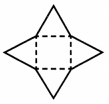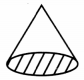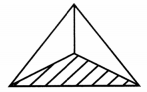# MCQ Questions for Class 7 Maths Chapter 15 Visualising Solid Shapes with Answers

We have compiled the NCERT MCQ Questions for Class 7 Maths Chapter 15 Visualising Solid Shapes with Answers Pdf free download covering the entire syllabus. Practice MCQ Questions for Class 7 Maths with Answers on a daily basis and score well in exams. Refer to the Visualising Solid Shapes Class 7 MCQs Questions with Answers here along with a detailed explanation.

## Visualising Solid Shapes Class 7 MCQs Questions with Answers

Choose the correct option.

Question 1.
By folding the adjoining net, we get(a) cube
(b) Cylinder
(c) A pyramid
(d) A cone

Question 2.
Which of the following is three dimensional?
(a) A plane
(b) A curve
(c) Cuboid
(d) A prism

Question 3.
Number of edges in a square pyramid is
(a) 6
(b) 8
(c) 10
(d) 12

Question 4.(a) Cone
(b) Square pyramid
(c) Prism
(d) Triangular pyramid

Question 5.
Which of the following solids has only one face?
(a) Cone
(b) Cylinder
(c) Sphere
(d) Pyramid

Question 6.
The name of the solid shape is(a) cylinder
(b) cone
(c) cuboid
(d) sphere

Question 7.
The number of vertices of the solid shape is(a) 1
(b) 2
(c) 3
(d) 4

Question 8.
The number of edges of the solid shapes(a) 1
(b) 2
(c) 3
(d) 6

Question 9.
What cross-section do you get when you give a horizontal cut to a die?
(a) Square
(b) Rectangle
(c) Triangle
(d) Circle

Question 10.
What cross-section do you get when you give a horizontal cut to a round apple?
(a) Circle
(b) Square
(c) Rectangle
(d) Triangle.

Question 11.
By folding the adjoining net, we get:
(a) A cube
(b) A cylinder
(c) A pyramid
(d) A cone

Question 12.
Which of the following is zero dimensional?
(a) A plane
(b) A print
(c) A curve
(d) A solid

Fill in the blanks

Question 1.
A square pyramid has ……………… faces.

Question 2.
A prism has ……………… edges.

Question 3.
The solid ……………… has no edges and no vertices.

Question 4.
The net for a cylinder without top and bottom is a ………………

Question 5.
A cone has ……………… curved surface.

Question 6.
A square pyramid has ………………… faces. [four/five]

Question 7.
A triangular pyramid has ………………… faces. [three/four]

Question 8.
The solid ………………… hast no edge and no vertex. [cylinder/sphere]

Question 9.
The net for a cylinder without top and bottom is a ………………… [rectangle/circle]

Question 10.
A cylinder has ………………… curved surface(s). [one/three]

Question 11.
A sphere has ………………… curved surface(s). [one/two]

Question 12.
A cylinder has ………………… vertex. [nil/one]

Question 13.
A sphere has ………………… vertex. [nil/one]

Match the following